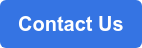# Four Steps to Take for

2022/07/14## How to Determine Sample Size: Four Steps You Need to Take for Your Company’s Market Research

Sampling is central to any kind of market research, and one aspect that you should take note of when you’re taking a sample is figuring out your sample size.

Why is sample size so important anyway? It’s because sample sizes are often what will determine whether your sample is representative. And, as we’ve mentioned in a previous article, representativeness is crucial in ensuring that your market research results truly reflect the condition that you are researching in your target population.

In this article, we’re going to guide you on how to determine sample size and use it to represent your researched population.

### What is sample size?

Sample size is the number of people or subjects that are used as a sample in market research. It differs from the number of samples - the latter means the number of times that you take a sample from the population.

So, if you take a sample three times in one research study, with each sample size being 25 people, your total sample size would be 75 people.

### How to find sample size?

There are many methods that researchers use to find the right sample size, but all of them can be boiled down into the following four steps:

#### Step 1 Find out the size of the population

The first step you’ll need to take is to find out exactly the number of people that comprises your population. For example, if your target population is men between the ages of 20-30, living in Singapore as citizens or permanent residents, you’ll need to use data from SingStat as your reference. From there, you can start calculating your sample size through several statistical methods, which we’ll discuss further below.

Nevertheless, there is a quick rule of thumb that you could use to determine sample size based on the size of the population. Here’s a table that GMO Research has prepared for you, based on our in-house technique:

Population size Estimated sample size
100 80
1,000 279
10,000 370
100,000 383
1,000,000 384
> 1,000,000 384

#### Step 2 Determine the margin of error

Now, if you’re interested in calculating your own sample size, there are two important statistical concepts that you’ll need to know: margin of error and confidence level. These two concepts are integral in determining sample size.

The margin of error, which we’re covering in this step, is basically measuring this: “How far does the survey result deviate from the actual population?"

What this means is this: If we use 1,000 people as the sample size to represent a population of 10,000,000 people, how dissimilar is the sample’s response to the population?

A good margin of error for market research is around 1-10%. The higher the percentage, the more the sample’s response does not resemble the population.

You’ll have to determine the margin of error that suffices for your market research study. Generally, we recommend no more than a 5% margin of error.

#### Step 3 Set confidence level

Confidence level refers to the probability that the sampling result is within the margin of error. A good confidence level ranges between 90-99%, but a market research study usually uses a 95% confidence level.

Here’s how confidence level works in practice: A 99% confidence level for a sample means that you are 99% certain that the response of your sample is reliable enough to describe the target population.

#### Step 4 Use a formula to find sample size

Now that you understand the concept of margin of error and confidence level, you can easily calculate sample size through this formula:• n = Sample size
• λ = Value based on confidence level
• p = Sample proportion
• d = Margin of error

There are two concepts we haven’t covered previously - the λ and p. These two are determined through statistical rules and conventions used in research studies.

For, λ (lambda), the numerical values are predetermined for each confidence level, and the frequently used ones are as follows.

Confidence level Value of λ
90% 1.65
95% 1.96
99% 2.58

Sample proportion (p) is determined by the previous research study since it calculates the ratio of the survey subjects who chose the relevant response.

Typically, you can set the sample proportion value at "50% (0.5 in the formula)" if you don’t have the data from previous research or pilot study.

##### Sample size calculation example

As an example, let's calculate the sample size under the following conditions.

• Margin of error: 4% (0.04)
• Confidence level: 99%
• Lambda: 2.58
• Sample proportion: 50% (0.5)From this formula, we can see that the sample size would be 1,040 people or subjects.

### Summary

Sample sizes are often the hallmark of representativeness, making this step extremely crucial in your market research journey. If you’re looking for assistance in determining the right sample size for your unique needs, contact us here:sample size how to find sample size what is sample size how to determine sample size margin of error confidence level